# High School Chemistry : Using Charles's Law

## Example Questions

### Example Question #8 : Gases And Gas Laws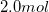of an ideal gas are contained in a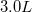container at a temperature of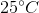. The gas exerts a pressure of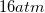on the container.

If pressure is kept constant, what is the final volume of the gas if the temperature of the container is increased to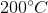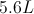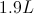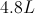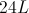Explanation:

Since pressure is kept constant, the only variable that is manipulated is temperature. This means that we can use Charles's law in order to compare volume and temperature. Since volume and temperature are on opposite sides of the ideal gas law, they are directly proportional to one another. As one variable increases, the other will increase as well.

Charles's law is written as follows: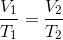To use this law, we must first convert the temperatures to Kelvin.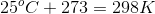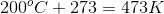Use these temperatures and the initial volume to solve for the final volume.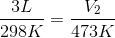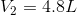### Example Question #1 : Gases And Gas Laws

Which law is the following formula?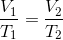Gay-Lussac's law

Boyle's law

Ideal gas law

Combined gas law

Charles's law

Charles's law

Explanation:

Charles's law defines the direct relationship between temperature and volume. When the parameters of a system change, Charles's law helps us anticipate the effect the changes have on volume and temperature.Boyle's law relates pressure and volume: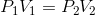Charles's law relates temperature and volume:Gay-Lussac's law relates temperature and pressure: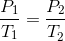The combined gas law takes Boyle's, Charles's, and Gay-Lussac's law and combines it into one law: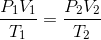The ideal gas law relates temperature, pressure, volume, and moles in coordination with the ideal gas constant: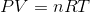### Example Question #10 : Gases And Gas Laws

A canister of gas has a volume of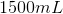at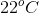. What volume will the gas occupy at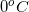if the pressure remains constant?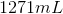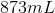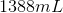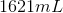Explanation:

Charles's law of gases indicates that, at a constant pressure, the volume of a gas is proportional to the temperature. This is calculated by the following equation:Our first step to solving this equation will be to convert the given temperatures to Kelvin.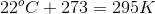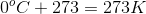Using these temperatures and the initial volume, we can solve for the final volume of the gas.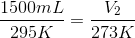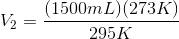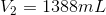### Example Question #1 : Using Charles's Law

A gas occupies a volume of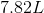at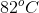. At what temperature, in Kelvin, will the volume of the gas be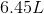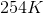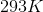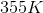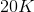Explanation:

Charles's law of gases indicates that, at a constant pressure, the volume of a gas is proportional to the temperature. This is calculated by the following equation:Our first step to solving this equation will be to convert the given temperature to Kelvin.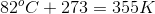Using this temperature and the given volumes, we can solve for the final temperature of the gas.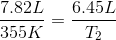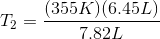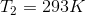### Example Question #1 : Using Charles's Law

The graph depicted below represents which of the gas laws?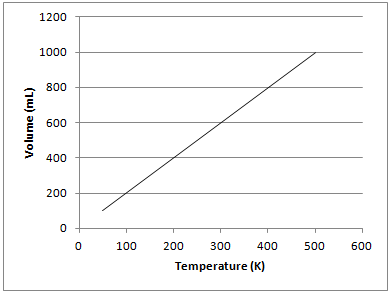Gay-Lussac's law

Charles's law

Newton's third law

Boyle's law

Charles's law

Explanation:

The graph shows that there is a directly proportional relationship between the volume of a gas and temperature in Kelvin when kept at a constant pressure. This is known as Charles’s law and can be represented mathematically as follows:Gay-Lussac's law shows the relationship between pressure and temperature. Boyle's law shows the relationship between pressure and volume. Newton's third law is not related to gas principles and states that for every force on an object, there is an equal and opposite force of the object on the source of force.

### Example Question #3 : Using Charles's Law

A balloon filled with room temperature air (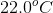) has a volume of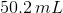. The balloon is taken outside on a hot summer day where the temperature is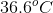. What will the volume of the balloon be after it is taken outside?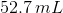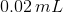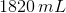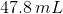Explanation:

We expect the volume to increase since volume and temperature are directly proportional. We know that if we heat something the material will expand so we shouldn't get a value that is smaller than our initial volume. Charles Law says thatwhere the stuff on the left is the initial volume and temperature and the stuff on the right is the final volume and temperature. First off, we MUST convert the temperatures to Kelvin to use Charles Law. This gives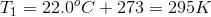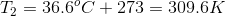Solving for the final volume,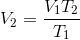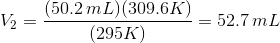### All High School Chemistry Resources